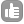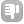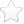0Hello, I run DOSY expt successfully but my dosy files are very massive and they take up large amounts of disk space. How can I reduce size of dosy files? Is it possible to select a small region around the peaks and do process only on that region? How? Thank you Elham asked Dec 08 '14 at 00:491Hi, probably you're conducting your experiments with utilization of excessive digital resolution (points per Hz). For example, one can consider 2D COSY spectrum obtained with 2048 points in the direct dimension (TD=2k) and 1024 increments in the indirect dimension (TD1=1k) with real size of the spectrum of 1024 points in each dimension (SI = SI1 =1k), as an outcome one obtains matrix of experimental data that consists 1024^2 elements (2nd order tensor). Each one of these matrix entries needs 8 bytes to be digitally described. Now it's time to do some math: ``````File_Size = (1024*1024*8) Bytes = [(2^10*(2^10)*(2^3)] Bytes = = (2^23)Bytes = (8388608) Bytes = 8 MBytes `````` Outcome of this calculation is the exact size of the *.ser file. One can calculate it for all types of 2D spectra and outcome always will be in the form of 2^n Bytes. File sizes of higher dimensional spectra can be calculated analogously. So if one wants to obtain for example 2D spectrum with final 8192 points in direct dimension and 8192 in indirect dimension (TD=16k, TD1=4k, SI=SI1=8k), must have in mind that file size will be: ``````File_Size = [(2^13)*(2^13)*8] Bytes = (2^29) Bytes = = (536870912) Bytes = 512 MB !!! `````` Algorithm for Fast Fourier Transformation (FFT) operates on DFT (Discrete Fourier Transformation) matrix so the more massive matrix is, the more computational power it requires and that equals, among other things, to long processing times of the spectrum. I don't know which variant of DOSY you're using, but I presume that is casual 2D DOSY. Probably the cause of your problem is a wrong setting of a few parameters : (Bruker TopSpin parameters) aqcupars: TD, TD1, AQ, DW, SW, SW1 procpars: SI, SI1, Linear Prediction parameters. Please paste these mentioned above parameters of your DOSY experiments and maybe we'll be able to help you (add also spectrometer 1H operating frequence). Personally, I don't know any solution of the unusual methods that could allow to process only fragments of 2D spectrum. Some sophisticated methods have been introduced to high dimensional spectra processing with nonuniform sampling. If you want to reduce the size of your files, you should in the first place choose the proper carrier wave frequency (SW) and adjust the spectral resolution settings ( TD, SI, AQ, DW) appropriately to your needs. Best regards, Arkadiusz Leniak answered Dec 08 '14 at 08:17Dear Arkadiusz Leniak,thanks for complete answer. acquisition parameters are: TD=32768 and 16(for F2) AQ=1.5860696 sec DW=48.4 usec SWH=10330.58 Hz SW=20.6557 ppm - elham (Dec 13 '14 at 08:10) please give me also pulse program name of your experiment. - Arkadiusz Leniak (Dec 15 '14 at 00:29) pulse program is ledbpgp2s. - elham (Dec 15 '14 at 04:37) I don't know what's wrong, but your file size should be c.a 4Mb. You should have matrix of 32k x 16 points. 16 transients with different gradient values (auto optimized by AU program). What size is your *.ser file ? - Arkadiusz Leniak (Dec 15 '14 at 13:42) size of my dosy file is 1.4Gb! how can I find size of *.ser file? - elham (Dec 22 '14 at 23:45)0Dear Arkadiusz Leniak,thanks for complete answer. acquisition parameters are: TD=32768 and 16(for F2) AQ=1.5860696 sec DW=48.4 usec SWH=10330.58 Hz SW=20.6557 ppm answered Dec 14 '14 at 22:390Dear Elham, It looks like your problem is in processing rather than acquisition. As Arkadiusz pointed out your files should have been much smaller. I am suspecting that you are processing your pseudo2D file with 512-1k points in the indirect dimension while you only need to use 16 as there is no use to zero fill your data in pseudo 2D data. Best Regards and happy new year. Manolis answered Jan 14 '15 at 07:53thank you dear Manolis. I'd like to know more about zero-filling data specially in the case of dosy experiment. As I found out from your answer I don't need to use zero filling. How can I apply this point during the dosy experiment? - elham (Jan 16 '15 at 07:57)